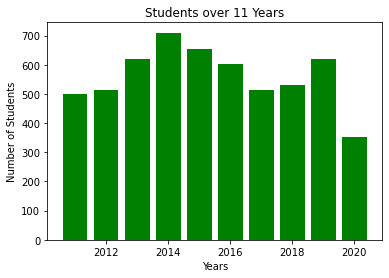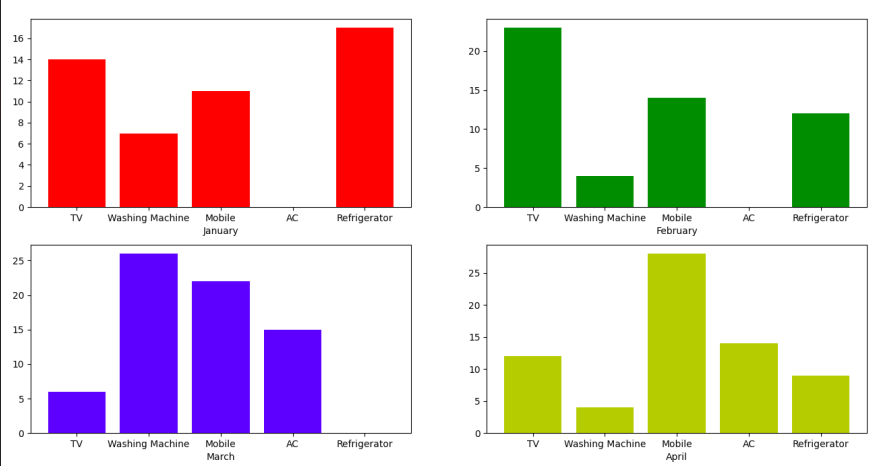Related Articles
How to plot Bar Graph in Python using CSV file?
• Difficulty Level : Hard
• Last Updated : 25 Feb, 2021

CSV stands for “comma separated values”, that means the values are distinguished by putting commas and newline characters. A CSV file provides a table like format that can be read by almost every spreadsheet reader like Microsoft Excel and Google Spreadsheet.

A Bar Graph uses labels and values where label is the name of a particular bar and value represent the height of the bar. A Bar Graph is commonly used in data analytics where we want to compare the data and extract the most common or highest groups.

In this post, we will learn how to plot a bar graph using a CSV file. There are plenty of modules available to read a .csv file like csv, pandas, etc. But in this post we will manually read the .csv file to get an idea of how things work.

### Functions Used

• Pandas read_csv() function is used to read a csv file.

Syntax:

read_csv(“file path”)

• Matplotlib’s bar() function is used to create a bar graph

Syntax:

plt.bar(x, height, width, bottom, align)

### Method 1: Using pandas

Approach

• Import module
• Read file using read_csv() function
• Plot bar graph
• Display graph

Example:

Dataset in use: Click here

## Python3

 `# Import the necessary modules``import` `matplotlib.pyplot as plt``import` `pandas as pd`` ` ` ` `# Initialize the lists for X and Y``data ``=` `pd.read_csv(``'C:\\Users\\Vanshi\\Desktop\\data.csv'``)`` ` `df ``=` `pd.DataFrame(data)`` ` `X ``=` `list``(df.iloc[:, ``0``])``Y ``=` `list``(df.iloc[:, ``1``])`` ` `# Plot the data using bar() method``plt.bar(X, Y, color``=``'g'``)``plt.title(``"Students over 11 Years"``)``plt.xlabel(``"Years"``)``plt.ylabel(``"Number of Students"``)`` ` `# Show the plot``plt.show()`

Output:### Method 2: Using Matplotlib

Approach

• Import module
• Open file
• Read data
• Plot bar graph
• Display graph

Program:

Dataset in use: Click here to download this file.

## Python3

 `# Import the modules``import` `matplotlib.pyplot as plt`` ` `# Initialize a dictionary for months``data ``=` `dict``()`` ` `# Read the data``with ``open``(``'electronics.csv'``, ``'r'``) as f:``    ``for` `line ``in` `f.readlines():``       ` `        ``# Store each line in the dictionary``        ``month, item, quantity ``=` `line.split(``','``)``         ` `        ``if` `month ``not` `in` `data:``            ``data[month] ``=` `[]``        ``data[month].append((item, ``int``(quantity)))`` ` `# Position of each subplot where 221 means 2 row,``# 2 columns, 1st index``positions ``=` `[``221``, ``222``, ``223``, ``224``]`` ` `# Colors to distinguish the plot``colors ``=` `[``'r'``, ``'g'``, ``'b'``, ``'y'``]`` ` `# Plot the subgraphs``for` `i, l ``in` `enumerate``(data.keys()):``    ``plt.subplot(positions[i])``    ``data_i ``=` `dict``(data[l])``    ``plt.bar(data_i.keys(), data_i.values(), color``=``colors[i])``    ``plt.xlabel(l)`` ` `# Show the plots``plt.show()`

Output:Attention geek! Strengthen your foundations with the Python Programming Foundation Course and learn the basics.

To begin with, your interview preparations Enhance your Data Structures concepts with the Python DS Course.

My Personal Notes arrow_drop_up